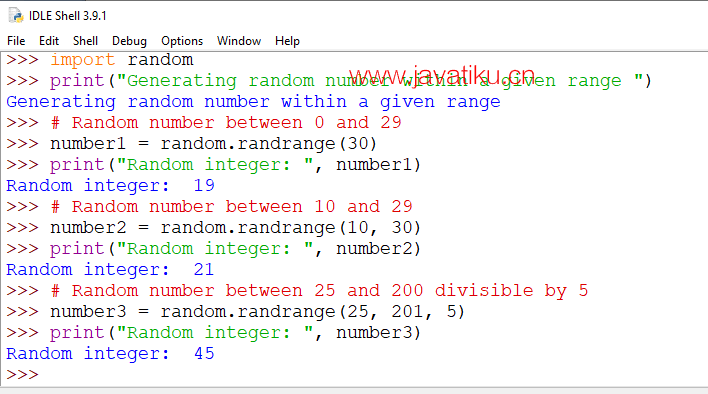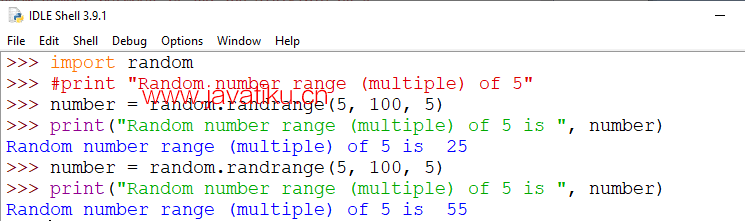# Tkinter 教程-Python随机数randrange()Python的random模块允许生成随机数。生成的数字是伪随机数序列，它们基于所使用的函数。在random模块中有不同类型的函数用于生成随机数，如random.random()，random.randint()，random.choice()，random.randrange(start, stop, width)等等。

## random.randrange()的语法

``random.randrange(start, stop[, width])``

random.randrange()函数返回给定范围内的随机整数。random.randrange()函数接受三个参数作为输入：start，stopwidth。在这三个参数中，两个参数startwidth是可选的。

• start参数是随机范围中的起始或下限整数。如果没有传递start参数，它将采用默认值0（零）。
• stop参数是随机范围中的最后一个数字或上限整数。
• width参数是在随机序列中的每个数字之间的范围。此参数是可选的，如果没有传递width参数，它将采用默认值1（一）。

randrange(start, stop, width)函数在生成随机整数时不包括结束参数。stop参数是排除的，它不会在随机数中生成。

### 示例1：random.randrange()生成给定范围内的随机整数

``````import random
print("Generating random number within a given range ")
# Random number between 0 and 29
number1 = random.randrange(30)
print("Random integer: ", number1)
# Random number between 10 and 29
number2 = random.randrange(10, 30)
print("Random integer: ", number2)
# Random number between 25 and 200 divisible by 5
number3 = random.randrange(25, 201, 5)
print("Random integer: ", number3)  ``````### 示例2：生成n的随机整数范围（多个）

``````import random
#print "Random number range (multiple) of 5"
number = random.randrange(5, 100, 5)
print("Random number range (multiple) of 5 is ", number)  ``````### 示例3：生成特定长度的随机整数

``````import random
number1 = random.randrange(100, 1000)
print("First random number of length 3 is: ", number1)
number2 = random.randrange(100, 1000)
print("Second random number of length 3 is: ", number2)  ``````

``````First random number of length 3 is: 813
Second random number of length 3 is: 770``````

### 示例4：生成随机负整数:

``````import random
randomNagativeInteger = random.randrange(-50, -10)
print("Random negative integer number between -50 to -10")
print("Random negative integer number between -50 to -10 is: ",randomNagativeInteger)  ``````

``````Random negative integer number between -50 to -10
Random negative integer number between -50 to -10 is:  -43``````

### 示例5：生成随机正整数或负整数

``````import random
number1 = random.randrange(-5, 5)
print(number1)
number2 = random.randrange(-5, 5)
print(number2)``````

``````-5
0``````

``````import random
randomNumber = random.randrange(10,5) ``````Python的random模块的一些其他函数，用于随机生成数字的包括：

• choice()
• randint()
• sample()
• sort()

## random.choice()

Python的random模块有一个choice()函数，用于从列表或其他序列中选择一个随机元素。random.choice()函数用于从值列表中返回一个随机元素。

random.choice()的语法

``random.choice(sequence)  ``

``random.choices(sequence, k=l)  ``

random.choices()函数中，k是要返回的元素数量。假设我们不指定k参数的值，random.choices()将返回序列或列表中的单个项目。

``````import random
listValue = [5,10,15,20,25,30]
item = random.choice(listValue)
print("random item from list is: ", item) ``````

``random item from list is:  10``

random.choices()函数主要用于返回具有不同可能性的随机元素。此函数还接受权重（k）的随机选择。例如，我们想要从5个电影的列表中随机选择3个电影名称。

``````import random
movieList = ['Matrix', 'Terminator', 'Black Panther', 'Avengers', 'Titanic']
randomMovies = random.choices(movieList, k=3)
print("random movies from list are: ", randomMovies) ``````

``random movies from list are:  ['Avengers', 'Black Panther', 'Titanic']``

### random.randint()

Python的random.randint()函数用于在指定范围内生成随机整数。

``random.randint(start, stop)``

random.randint()函数接受两个参数start，它是起始范围，以及stop，它是结束范围。在生成随机数时，这两个参数（start, stop）都包括在内。

``````import random
randomListValue = []
# specifing length of list equal to 5
for i in range(0, 5):
# generates random numbers from 1 to 100
randomListValue.append(random.randint(1, 100))
print("Printing list of 5 generated random numbers")
print(randomListValue)``````

``````Printing list of 5 generated random numbers
[65, 82, 3, 39, 40]``````

## random.sample()

random.sample()函数从集合、列表和其他序列中生成唯一元素。Python的random.sample()函数允许从列表中随机抽样多个元素，而不会重复。

``````import random
# Generate 10 unique random numbers within a range
randomList = random.sample(range(0, 100), 10)
print(randomList) ``````

``[15, 17, 16, 66, 34, 85, 71, 82, 97, 48]``

``````import random
# Generate 10 unique random numbers within a range
randomList = random.sample(range(0, 100, 5), 10)
print(randomList) ``````

``[75, 40, 20, 55, 15, 10, 5, 90, 95, 70]``

## 列表排序

Python的sort()函数用于按升序（默认情况下）对列表中的随机整数进行排序。我们还可以通过指定其reverse = True参数来按降序对列表值进行排序。reverse的默认值为False

``````import random
randomList = random.sample(range(50, 100, 5), 5)
print("Before sorting random integers list")
print(randomList)
# sort the list ascending order by default
randomList.sort()
print("After sorting random integers list")
print(randomList)``````

``````Before sorting random integers list
[90, 80, 60, 55, 85]
After sorting random integers list
[55, 60, 80, 85, 90]``````

``````import random
randomList = random.sample(range(50, 100, 5), 5)
print("Before sorting random integers list")
print(randomList)
# sort the list descending order
randomList.sort(reverse=True)
print("After sorting random integers list")
print(randomList)  ``````

``````Before sorting random integers list
[70, 50, 80, 90, 85]
After sorting random integers list
[90, 85, 80, 70, 50]``````